Courses

# The Direct Stiffness Method: Beams - 6 GATE Notes | EduRev

## GATE : The Direct Stiffness Method: Beams - 6 GATE Notes | EduRev

The document The Direct Stiffness Method: Beams - 6 GATE Notes | EduRev is a part of the GATE Course Structural Analysis.
All you need of GATE at this link: GATE

Instructional Objectives

After reading this chapter the student will be able to

1. Compute moments developed in the continuous beam due to support settlements.
2. Compute moments developed in statically indeterminate beams due to temperature changes.
3. Analyse continuous beam subjected to temperature changes and support settlements.

Introduction

In the last two lessons, the analysis of continuous beam by direct stiffness matrix method is discussed. It is assumed in the analysis that the supports are unyielding and the temperature is maintained constant. However, support settlements can never be prevented altogether and hence it is necessary to make provisions in design for future unequal vertical settlements of supports and probable rotations of fixed supports. The effect of temperature changes and support settlements can easily be incorporated in the direct stiffness method and is discussed in this lesson. Both temperature changes and support settlements induce fixed end actions in the restrained beams. These fixed end forces are handled in the same way as those due to loads on the members in the analysis. In other words, the global load vector is formulated by considering fixed end actions due to both support settlements and external loads. At the end, a few problems are solved to illustrate the procedure.

Support settlements

Consider continuous beam ABC as shown in Fig. 29.1a. Assume that the flexural rigidity of the continuous beam is constant throughout. Let the support B settles by an amount Δ as shown in the figure. The fixed end actions due to loads are shown in Fig. 29.1b. The support settlements also induce fixed end actions and are shown in Fig. 29.1c. In Fig. 29.1d, the equivalent joint loads are shown. Since the beam is restrained against displacement in Fig. 29.1b and Fig. 29.1c, the displacements produced in the beam by the joint loads in Fig. 29.1d must be equal to the displacement produced in the beam by the actual loads in Fig. 29.1a. Thus to incorporate the effect of support settlement in the analysis it is required to modify the load vector by considering the negative of the fixed end actions acting on the restrained beam.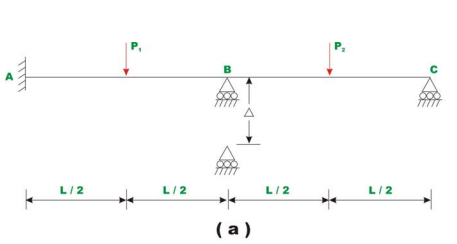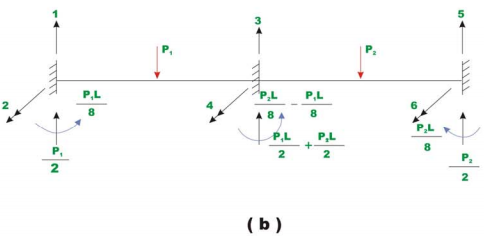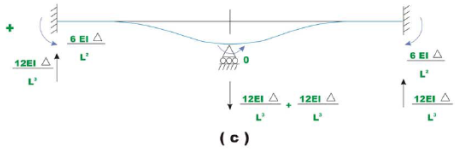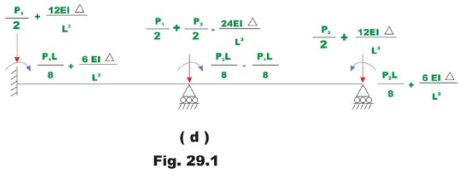Effect of temperature change

The effect of temperature on the statically indeterminate beams has already been discussed in lesson 9 of module 2 in connection with the flexibility matrix method. Consider the continuous beam ABC as shown in Fig. 29.2a, in which span BC is subjected to a differential temperature T1 at top and T2 at the bottom of the beam. Let temperature in span AB be constant. Let D be the depth of beam and EI be the flexural rigidity. As the cross section of the member remains plane after bending, the relative angle of rotation dθ between two cross sections at a distance dx apart is given by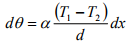(29.1)

where α is the co-efficient of the thermal expansion of the material. When beam is restrained, the temperature change induces fixed end moments in the beam as shown in Fig. 29.2b. The fixed end moments developed are,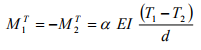(29.2)

Corresponding to the above fixed end moments; the equivalent joint loads can easily be constructed. Also due to differential temperatures there will not be any vertical forces/reactions in the beam.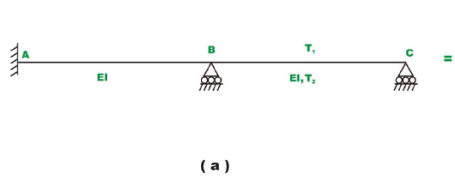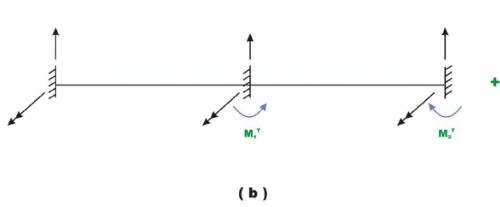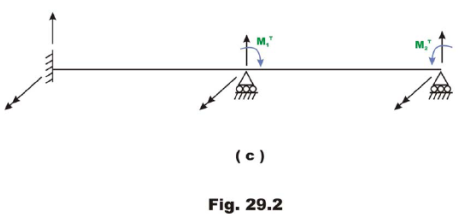Example 29.1

Calculate support reactions in the continuous beam ABC (vide Fig. 29.3a) having constant flexural rigidity EI , throughout due to vertical settlement of support B , by 5mm as shown in the figure. Assume E = 200 GPa and I = 4 × 10-4 10 m4 .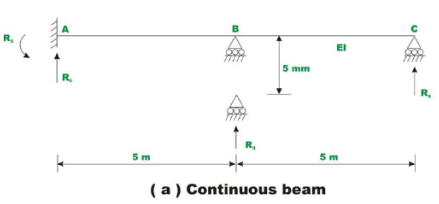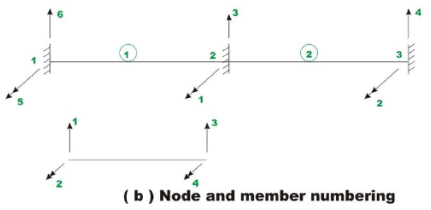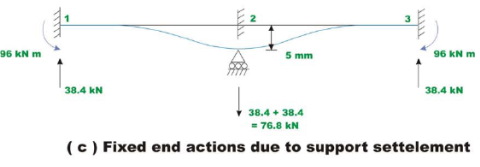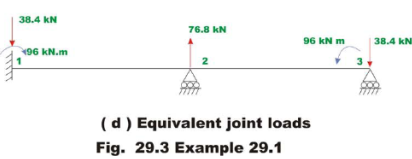The continuous beam considered is divided into two beam elements. The numbering of the joints and members are shown in Fig. 29.3b. The possible global degrees of freedom are also shown in the figure. A typical beam element with two degrees of freedom at each node is also shown in the figure. For this problem, the unconstrained degrees of freedom are 1u and 2u . The fixed end actions due to support settlement are,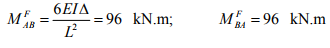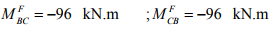(1)

The fixed-end moments due to support settlements are shown in Fig. 29.3c.

The equivalent joint loads due to support settlement are shown in Fig. 29.3d. In the next step, let us construct member stiffness matrix for each member.

Member 1: L = 5m , node points 1-2.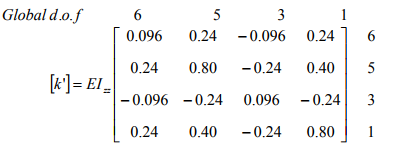(2)

Member 2: L = 5m , node points 2-3.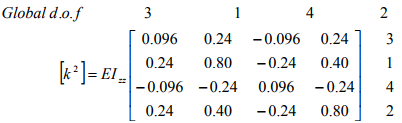(3)

On the member stiffness matrix, the corresponding global degrees of freedom are indicated to facilitate assembling. The assembled global stiffness matrix is of order 6×6. Assembled stiffness matrix [K]is given by,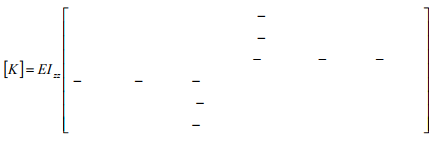(4)

Thus the global load vector corresponding to unconstrained degrees of freedom is,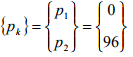(5)

Thus the load displacement relation for the entire continuous beam is,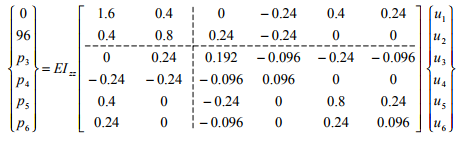(6)

Since, u3 = u4 = u5 = u6 = 0 due to support conditions. We get,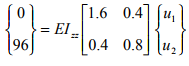Thus solving for unknowns u1 and u2 ,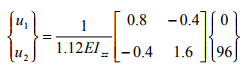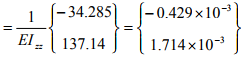u1 =-0.429 x 10-3 radians;    u2 = 1.714 x 10-3 radians                         (7)

Now, unknown joint loads are calculated by,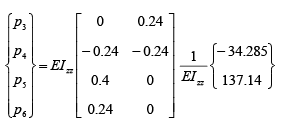(8)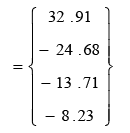Now the actual support reactions R3 , R4 , R5 and R6 must include the fixed end support reactions. Thus,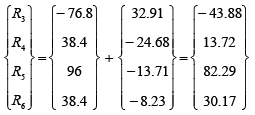(9)

R3 =-43.88 kN; R4 = 13.72 kN;    R5 = 82.29 kN.m; R6 = 30.17 kN                (10)

Offer running on EduRev: Apply code STAYHOME200 to get INR 200 off on our premium plan EduRev Infinity!

## Structural Analysis

30 videos|122 docs|28 tests

,

,

,

,

,

,

,

,

,

,

,

,

,

,

,

,

,

,

,

,

,

;